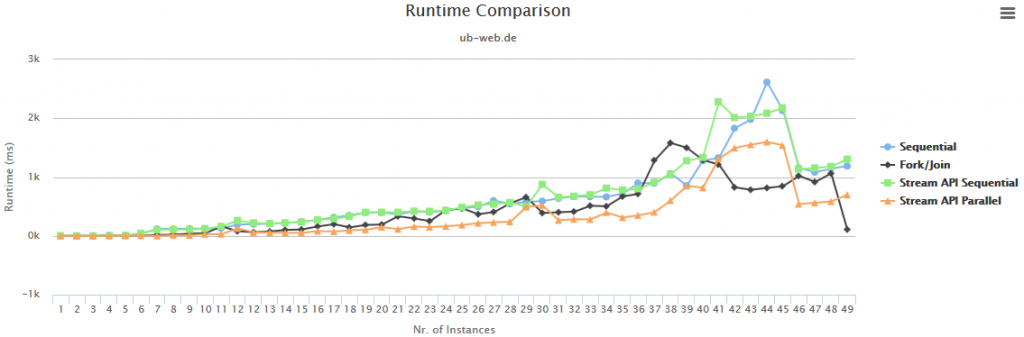# Java Parallelization

Created: 19.09.2014

## The Sample

I use generated text documents to ensure a similar setting for all scenarios. These text documents contain random generated numbers between 0 - 10.000 as replacement for words. Each document contains 200.000 space separated numbers. All of them are allready loaded into memory, no hard drive access is needed.

The corresponding text processing task is simple: tokenize these files. I simulate each approach for 50 rounds. While round 1 consists of only one file, round 50 consists of 50 files. To minimize random effects, i simulate each round 100 times and average the runtime values. My system runs on an Intel i5 with 4 cores.

## The Baseline: Sequential Processing

The processing unit looks like this:

``````timeMeasurements = new HashMap<>();
for (int nrOfDocs = 1; nrOfDocs < (NR_OF_RUNS + 1); nrOfDocs++) {
for(int i = 0; i < NR_OF_REPLICATIONS; i++){
long startTime = System.currentTimeMillis();
for (TextDocument document : documents) {
document.setTokenizedContent(document.getContent().split("\\s"));
}
long endTime = System.currentTimeMillis();
Long oldTime = timeMeasurements.get(nrOfDocs);
if(oldTime != null){
timeMeasurements.put(nrOfDocs, oldTime + ((endTime - startTime) / NR_OF_REPLICATIONS));
} else {
timeMeasurements.put(nrOfDocs, (endTime - startTime) / NR_OF_REPLICATIONS);
}
}
}``````

As you can see, i use the String.split() method to perform the tokenization. I avoided StringTokenizer since it is marked as deprecated.

## Parallel Processing with the Fork/Join Framework

The Fork/Join Framework is well suited for tasks that can be modeled as divide and conquer problems. Text processing often transforms a list of documents into a list of modified documents. Each document can be processed on its own without side effects. That means the list can be split up into smaller parts and processed by several threads.

Such a program could look like this:

``````timeMeasurements = new HashMap<>();
for (int nrOfDocs = 1; nrOfDocs < (NR_OF_RUNS + 1); nrOfDocs++) {
for(int i = 0; i < NR_OF_REPLICATIONS; i++){
long startTime = System.currentTimeMillis();
long endTime = System.currentTimeMillis();
Long oldTime = timeMeasurements.get(nrOfDocs);
if(oldTime != null){
timeMeasurements.put(nrOfDocs, oldTime + ((endTime - startTime) / NR_OF_REPLICATIONS));
} else {
timeMeasurements.put(nrOfDocs, (endTime - startTime) / NR_OF_REPLICATIONS);
}
forkPool.shutdown();
}
}``````

While the corresponding ProcessDocumentsTask looks like this:

``````public class ProcessDocumentsTask extends RecursiveTask<Void> {
private static final long serialVersionUID = 1L;
private List<TextDocument> documents;
private int from;
private int to;
private int range;

public ProcessDocumentsTask(List<TextDocument> documents, int range, int from, int to) {
this.documents = documents;
this.range = range;
this.from = from;
this.to = to;
}

@Override
protected Void compute() {
if (to - from <= range) {
TextDocument document;

for (int i = from; i <= to; i++) {
document = documents.get(i);
document.setTokenizedContent(document.getContent().split("\\s"));
}
} else {
int mid = (from + to) / 2;
firstHalf.fork();
secondHalf.compute();
firstHalf.join();
}
return null;
}
}``````

The value of NUM_THREADS is hardcoded and based on the number of cores i got on my machine (4 in this case). You can also programatically determine the value by asking the System class.

## Streaming API

The Streaming API comes in two flavours: sequential and parallel processing. Both flavours are built-in and easy-to-use as the following two code snippets demonstrate:

Sequential processing:

``````timeMeasurements = new HashMap<>();
for (int nrOfDocs = 1; nrOfDocs < (NR_OF_RUNS + 1); nrOfDocs++) {
for(int i = 0; i < NR_OF_REPLICATIONS; i++){
long startTime = System.currentTimeMillis();
documents.stream().forEach(textDocument ->
textDocument.setTokenizedContent(textDocument.getContent().split("\\s"))
);
long endTime = System.currentTimeMillis();
Long oldTime = timeMeasurements.get(nrOfDocs);
if(oldTime != null){
timeMeasurements.put(nrOfDocs, oldTime + ((endTime - startTime) / NR_OF_REPLICATIONS));
} else {
timeMeasurements.put(nrOfDocs, (endTime - startTime) / NR_OF_REPLICATIONS);
}
}
}``````

Parallel processing:

``documents.parallelStream().forEach(textDocument -> textDocument.setTokenizedContent(textDocument.getContent().split("\\s")))`;`

## Evaluation of the Approaches

To evaluate the approaches described above, i constructed a diagram depicting the number of documents processed on the x-axis and the time passed on the y-axis.The parallel Streaming API performs best on most of the scenarios. However, in some cases the Fork/Join Framework can achieve better results. Even though the last measurement might be an outlier, it performs well on the 40+ document scenarios. The sequential Streaming API and the naive sequential approach are both on par.